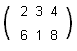HOME MATH DICTIONARY DOWNLOAD FEEDBACK DISCLAIMER
 Question: What is a Matrix ? Answer: A matrix is a set of numbers arranged in rows and columns in a rectangular or square array. The numbers and letters which make up the array are called its elements and can be referred to by giving their row and column. For example,The element in the second row and third column of the first matrix is 8. Matrices can be used to encrypt numerical data. Encryption is done by multiplying the data matrix with a key matrix. Decryption is done simply by multiplying the encrypted matrix with the inverse of the key.